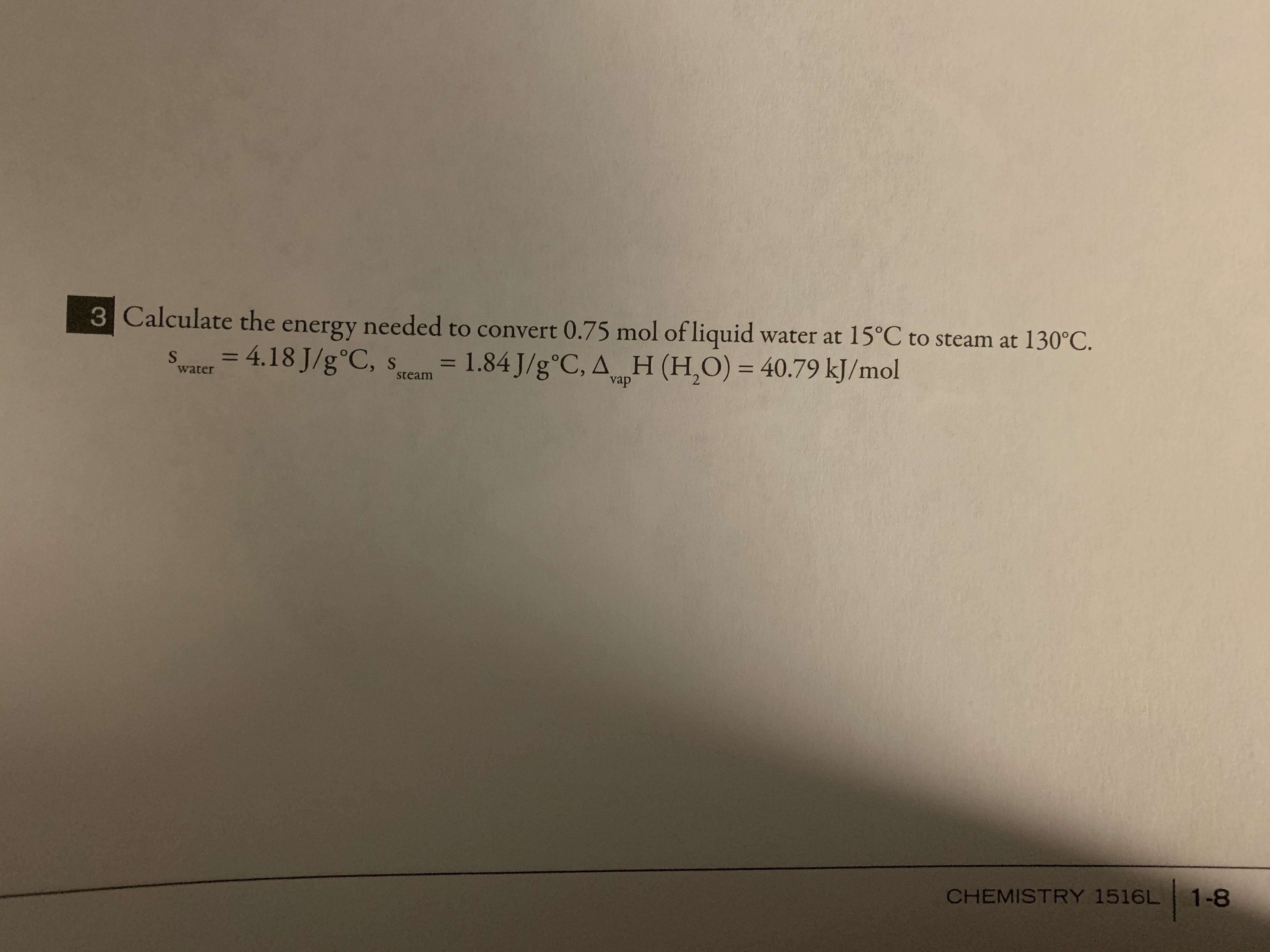# 3 Calculate the energy needed to convert 0.75 mol of liquid water at 15°C to steam at 130°C.= 4.18 J/g°C, s1.84 J/g°C, A H (H,O) = 40.79 kJ/mol%3D%3DwatersteamvapCHEMISTRY 1516L 1-8

Question
12 viewshelp_outlineImage Transcriptionclose3 Calculate the energy needed to convert 0.75 mol of liquid water at 15°C to steam at 130°C. = 4.18 J/g°C, s 1.84 J/g°C, A H (H,O) = 40.79 kJ/mol %3D %3D water steam vap CHEMISTRY 1516L 1-8 fullscreen
check_circle

Step 1

Given,

Moles of liquid water = 0.75 mol

Initial temperature of water = 15.0°C

Final temperature of steam = 130°C

Specific heat of water, swater = 4.184 J/g°C

Specific heat of steam, ssteam = 1.84 J/g°C

Heat of vaporisation, △vapH = 40.79 kJ/mol

Mass of water can be calculated as :

Step 2

The heat involved in this process can be written as :

Step 3

The value of q1 , q2 and q3 can be calc...

### Want to see the full answer?

See Solution

#### Want to see this answer and more?

Solutions are written by subject experts who are available 24/7. Questions are typically answered within 1 hour.*

See Solution
*Response times may vary by subject and question.
Tagged in

### Chemistry